# C++ Program to Calculate Average and Percentage Marks

Here you will learn and get code for finding the average and percentage mark of a student based on the marks entered by the user in C++. You will go through the following three programs over here:

### How Do I Determine the Average Mark?

Simply add all of the marks and divide by n to find the average of n subjects. For example, to find the average mark with marks obtained in three subjects, say 10, 20, and 30, then it will be:

```avg = (10+20+30)/3
= 60/3
= 20```

### How Do You Find a Percentage Mark?

To find the percentage mark, use the following formula:

`perc = ((marks obtained)/(total marks))*100`

For example, if three subjects have marks of 10, 11, and 12 out of 25, that is, the maximum score is 25. And out of 25, the student got 10, 11, and 12 in three subjects. Therefore, his or her percentage mark can be calculated as follows:

```perc = ((10+11+12)/(25*3))*100
= (33/75)*100
= (0.44)*100
= 44```

Therefore, the percentage mark will be 44 percent. Now let's move on to the program.

## In C++, Find the Average and Percentage Marks of Students

To calculate the average and percentage marks (in 5 subjects) of a student in C++ programming, you have to ask the user to enter marks obtained in 5 subjects.

In C++ programming, you have to ask the user to enter their marks in 5 subjects so you can figure out their average and percentage of marks in those 5 subjects.

Now place the summation result of 5 subjects' marks in a variable, say sum, and place sum/5 in a variable, say avg (the average of 5 subjects). Then place sum/500*100 in a variable named "perc" (percentage marks), and then display the result on the screen as shown here in the following program.

```#include<iostream>
using namespace std;
int main()
{
int i, mark;
float sum=0, avg, perc;
cout<<"Enter Marks obtained in 5 Subjects: ";
for(i=0; i<5; i++)
{
cin>>mark[i];
sum = sum+mark[i];
}
avg = sum/5;
perc = (sum/500)*100;
cout<<"\nAverage Marks = "<<avg;
cout<<"\nPercentage Marks = "<<perc<<"%";
cout<<endl;
return 0;
}```

This program was built and runs under the Code::Blocks IDE. Here is its sample run: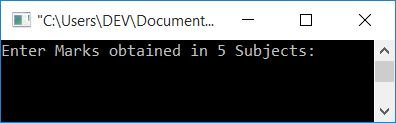Now supply the marks obtained in 5 subjects, say, physics, chemistry, math, English, and CS. Each mark should be entered out of 100. After supplying marks 78, 92, 83, 81, and 89, press the ENTER key to see the following output: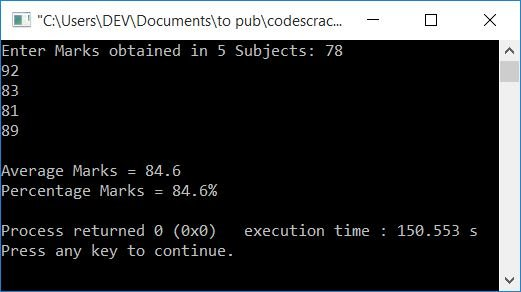### Allow the user to define the maximum mark

This program is identical to the previous one. But there are two extra features added to it. The first is that the user can enter the number of subjects, or how many subjects are currently running for his or her course. The second is that the user is permitted to enter the maximum possible mark. The rest will remain the same:

```#include<iostream>
using namespace std;
int main()
{
int i, n;
float tot, arr, sum=0, avg, perc;
cout<<"Enter Number of Subjects: ";
cin>>n;
cout<<"\nEnter Total Marks: ";
cin>>tot;
cout<<"\nEnter Marks obtained in "<<n<<" Subjects: ";
for(i=0; i<n; i++)
{
cin>>arr[i];
sum = sum+arr[i];
}
tot = tot*n;
avg = sum/n;
perc = (sum/tot)*100;
cout<<"\nAverage Marks = "<<avg;
cout<<"\nPercentage Marks = "<<perc<<"%";
cout<<endl;
return 0;
}```

Here is its sample run, with user input:

• 3 as the Number of Subjects
• 25 as Total Marks
• 10, 11, and 12 as Marks Obtained in 3 Subjects

Press the ENTER key after supplying the inputs as given above, and here is the output you will see: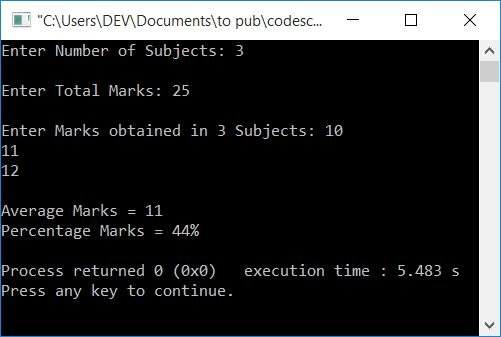### Using a user-defined function

This is the last program that also finds and prints averages and percentage marks. The only difference is that this program finds average and percentage marks using a function namelyaverageMark() and percMark().

```#include<iostream>
using namespace std;
float averageMark(float [], int);
float percMark(float [], int);
int main()
{
int i, n;
float arr, avg, per;
cout<<"Enter Number of Subjects: ";
cin>>n;
cout<<"\nEnter Marks obtained in "<<n<<" Subjects: ";
for(i=0; i<n; i++)
cin>>arr[i];
avg = averageMark(arr, n);
per = percMark(arr, n);
cout<<"\nAverage Marks = "<<avg;
cout<<"\nPercentage Marks = "<<per<<"%";
cout<<endl;
return 0;
}
float averageMark(float arr[], int n)
{
int i;
float sum=0;
for(i=0; i<n; i++)
sum = sum+arr[i];
return (sum/n);
}
float percMark(float arr[], int n)
{
float perc;
perc = averageMark(arr, n);
return perc;
}```

Here is its sample run: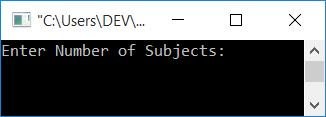Now enter the number of subjects running in your institute for your course. For example, if you're studying a course that includes six subjects, then enter 6. Since there are 6 subjects in your course, enter the marks obtained in all the 6 subjects (out of 100 each) and press the ENTER key to see the average and percentage marks as shown in the output given below: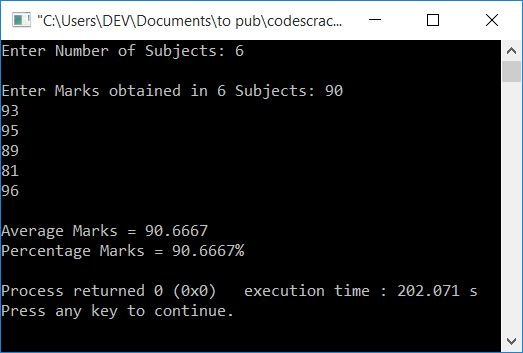#### The same program in different languages

C++ Quiz

« Previous Program Next Program »